# Samacheer Kalvi 11th Maths Solutions Chapter 4 Combinatorics and Mathematical Induction Ex 4.4

## Tamilnadu Samacheer Kalvi 11th Maths Solutions Chapter 4 Combinatorics and Mathematical Induction Ex 4.4

Question 1.
By the principle of mathematical induction, prove that, for n ≥ 1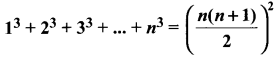Solution: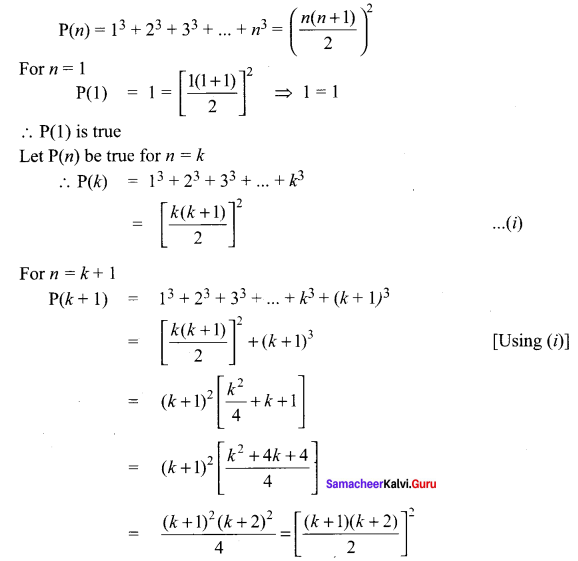∴ P(k+ 1) is true.
Thus P(K) is true ⇒ (k + 1) is true.
Hence by principle of mathematical induction, P(n) is true for all n ∈ N.

Question 2.
By the principle of mathematical induction, prove that, for n > 1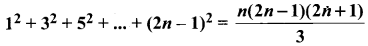Solution:∴ P(1) is true
Let P(n) be true for n = k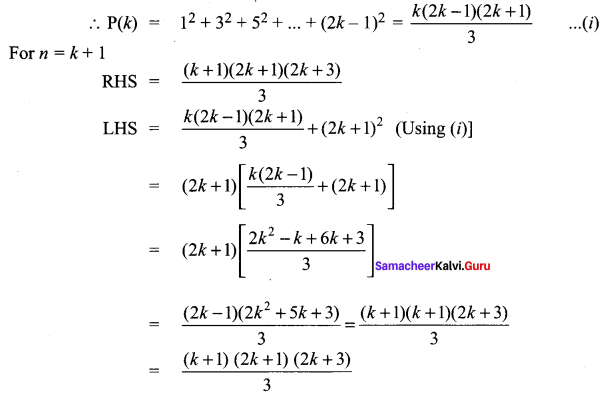∴ P(k + 1) is true
Thus P(k) is true ⇒ P(k + 1) is true. Hence by principle of mathematical induction, P(k) is true for all n ∈ N.Question 3.
Prove that the sum of the first n non-zero even numbers is n2 + n.
Solution:
Let P(n) : 2 + 4 + 6 +…+2n = n2 + n, ∀ n ∈ N

Step 1:
P( 1) : 2 = 12 + 1 = 2 which is true for P( 1)

Step 2:
P(k): 2 + 4 + 6+ …+ 2k = k2 + k. Let it be true.

Step 3:
P(k + 1) : 2 + 4 + 6 + … + 2k + (2k + 2)
= k2+ k + (2k + 2) = k2 + 3k + 2
= k2 + 2k + k + 1 + 1
= (k+ 1)2+ (k + 1)
Which is true for P(k + 1)
So, P(k + 1) is true whenever P(k) is true.

Question 4.
By the principle of Mathematical induction, prove that, for n ≥ 1.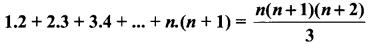Solution: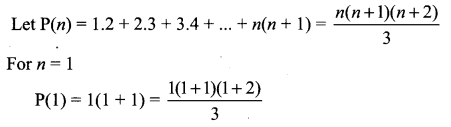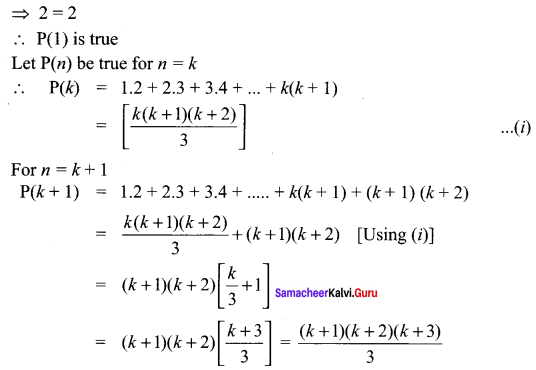∴ P(k + 1) is true
Thus P(k) is true ⇒ P(k + 1) is true
Hence by principle of mathematical induction, P(n) is true for all n ∈ N

Question 5.
Using the Mathematical induction, show that for any natural number n ≥ 2,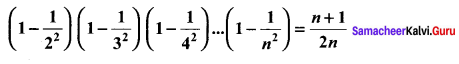Solution: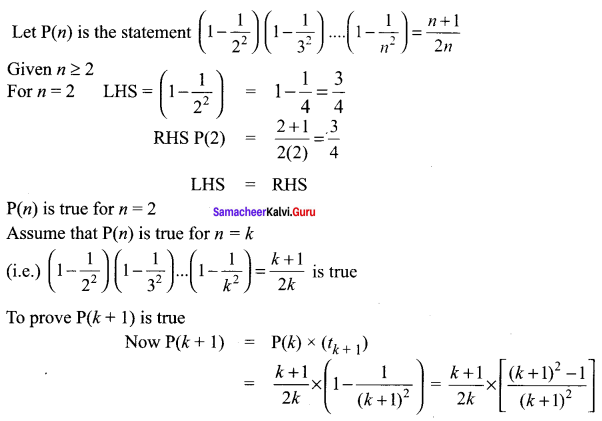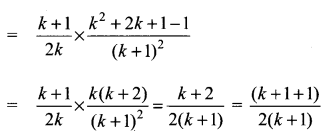⇒ P(k + 1) is true when P(k) is true so by the principle of mathematical induction P(n) is true.

Question 6.
Using the Mathematical induction, show that for any natural number n ≥ 2,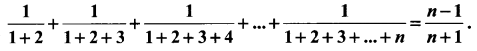Solution: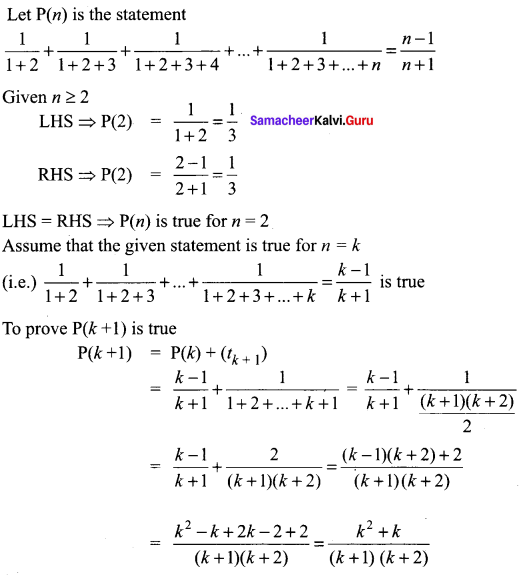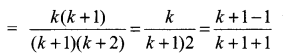⇒ P(k + 1) is true when P(k) is true so by the principle of mathematical induction P(n) is true for n ≥ 2.Question 7.
Using the Mathematical induction, show that for any natural number n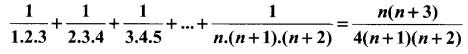Solution: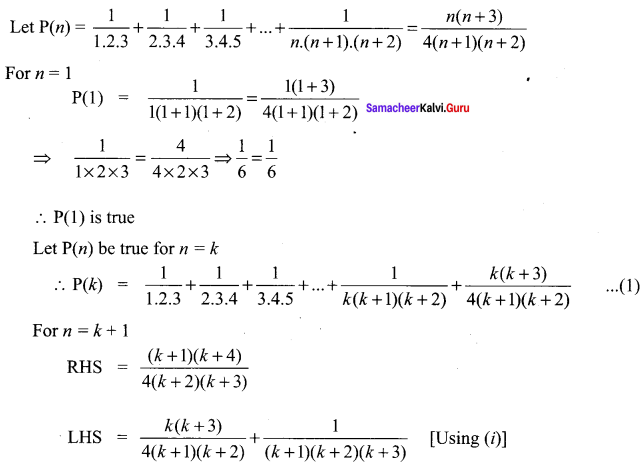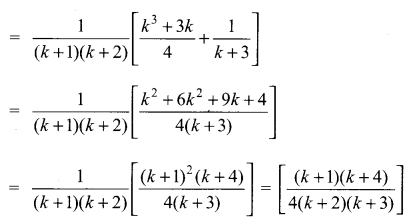∴ P(k + 1) is true
Thus p(k) is true ⇒ P(k + 1) is true
Hence by principle of mathematical induction,
p(n) is true for all n ∈ z

Question 8.
Using the Mathematical induction, show that for any natural number n,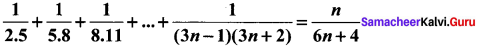Solution: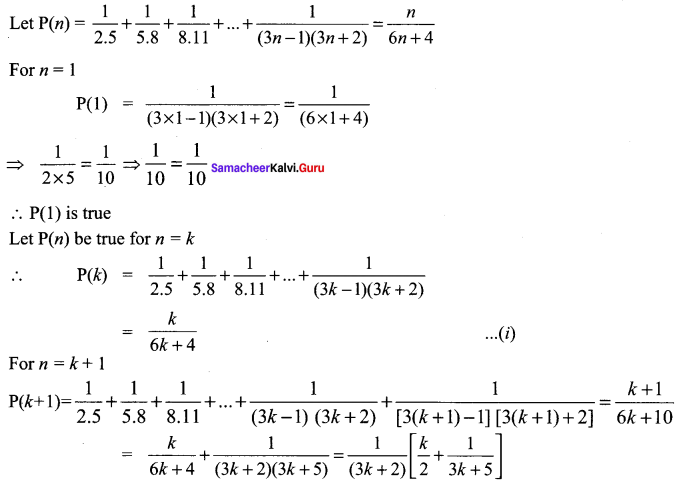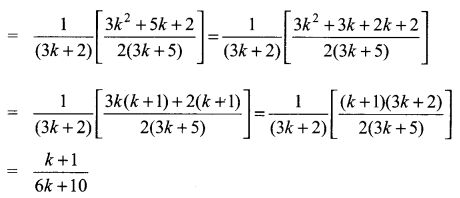∴ P(k + 1) is true
Thus P(k) is true ⇒ P(k + 1) is true. Hence by principle of mathematical induction, P(n) is true for all n ∈ N.Question 9.
Prove by Mathematical Induction that
1! + (2 × 2!) + (3 × 3!) + … + (n × n!) = (n + 1)! – 1
Solution:
P(n) is the statement
1! + (2 × 2!) + (3 × 3!) + ….. + (n × n!) = (n + 1)! – 1
To prove for n = 1
LHS = 1! = 1
RHS = (1 + 1)! – 1 = 2! – 1 = 2 – 1 = 1
LHS = RHS ⇒ P(1) is true
Assume that the given statement is true for n = k
(i.e.) 1! + (2 × 2!) + (3 × 3!) + … + (k × k!) = (k + 1)! – 1 is true
To prove P(k + 1) is true
p(k + 1) = p(k) + t(k + 1)
P(k + 1) = (k + 1)! – 1 + (k + 1) × (k + 1)!
= (k + 1)! + (k + 1) (k + 1)! – 1
= (k + 1)! [1 + k + 1] – 1
= (k + 1)! (k + 2) – 1
= (k + 2)! – 1
= (k + 1 + 1)! – 1
∴ P(k + 1) is true ⇒ P(k) is true, So by the principle of mathematical induction P(n) is true.

Question 10.
Using the Mathematical induction, show that for any natural number n, x2n – y2n is divisible by x +y.
Solution:
Let P(n) = x2n – y2n is divisible by (x + y)
For n = 1
P(1) = x2 × 1 – y2 × 1 is divisible by (x + y)
⇒ (x + y) (x – y) is divisible by (x + y)
∴ P(1) is true
Let P(n) be true for n = k
∴ P(k) = x2k – y2k is divisible by (x + y)
⇒ x2k – y2k = λ(x + y) …… (i)
For n = k + 1
⇒ P(k + 1) = x2(k + 1) – y2(k + 1) is divisible by (x + y)
Now x2(k + 2) – y2(k + 2)
= x2k + 2 – x2ky2 + x2ky2 – y2k + 2
= x2k.x2 – x2ky2 + x2ky2 – y2ky2
= x2k (x2 – y2) + y2λ (x + y) [Using (i)]
⇒ x2k + 2 – y2k + 2 is divisible by (x + y)
∴ P(k + 1) is true.
Thus P(k) is true ⇒ P(k + 1) is true. Hence by principle of mathematical induction, P(n) is true for all n ∈ NQuestion 11.
By the principle of mathematical induction, prove that, for n ≥ 1,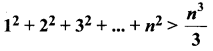Solution: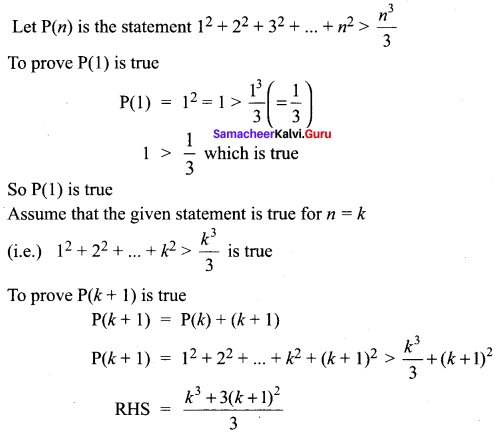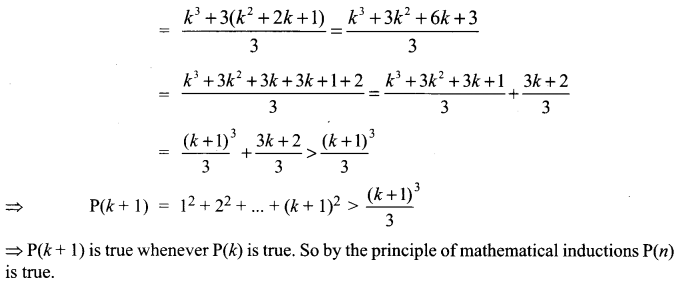Question 12.
Use induction to prove that n3 – 7n + 3, is divisible by 3, for all natural numbers n.
Solution:
Let P(n) : n3 – 7n + 3
Step 1:
P(1) = (1)3 – 7(1) + 3
= 1 – 7 + 3 = -3 which is divisible by 3
So, it is true for P(1).

Step 2:
P(k) : k3 – 7k + 3 = 3λ. Let it be true
⇒ k3 = 3λ + 7k – 3

Step 3:
P(k + 1) = (k + 1)3 – 7(k + 1) + 3
= k3 + 1 + 3k2 + 3k – 7k – 7 + 3
= k3 + 3k2 – 4k – 3
= (3λ + 7k – 3) + 3k2 – 4k – 3 (from Step 2)
= 3k2 + 3k + 3λ – 6
= 3(k2 + k + λ – 2) which is divisible by 3.
So it is true for P(k + 1).
Hence, P(k + 1) is true whenever it is true for P(k).

Question 13.
Use induction to prove that 5n + 1 + 4 × 6n when divided by 20 leaves a remainder 9, for all natural numbers n.
Solution:
P(n) is the statement 5n + 1 + 4 × 6n – 9 is ÷ by 20
P(1) = 51 + 1 + 4 × 61 – 9 = 52 + 24 – 9
= 25 + 24 – 9 = 40 ÷ by 20
So P(1) is true
Assume that the given statement is true for n = k
(i.e) 5k + 1 + 4 × 6n – 9 is ÷ by 20
P(1) = 51 + 1 + 4 × 61 – 9
= 25 + 24 – 9
So P(1) is true
To prove P(k + 1) is true
P(k + 1) = 5k + 1 + 1 + 4 × 6k + 1 + 1 – 9
= 5 × 5 k + 1 + 4 × 6 × 6k – 9
= 5[20C + 9 – 4 × 6k] + 24 × 6k – 9 [from(1)]
= 100C + 45 – 206k + 246k – 9
= 100C + 46k + 36
= 100C + 4(9 + 6k)
Now for k = 1 ⇒ 4(9 + 6k) = 4(9 + 6)
= 4 × 15 = 60 ÷ by 20 .
for k = 2 = 4(9 + 62) = 4 × 45 = 180 ÷ 20
So by the principle of mathematical induction 4(9 + 6k) is ÷ by 20
Now 100C is ÷ by 20.
So 100C + 4(9 + 6k) is ÷ by 20
⇒ P(k + 1) is true whenever P(k) is true. So by the principle of mathematical induction P(n) is true.Question 14.
Use induction to prove that 10n + 3 × 4n + 2 + 5, is divisible by 9, for all natural numbers n.
Solution:
P(n) is the statement 10n + 3 × 4n + 2 + 5 is ÷ by 9
P(1) = 101 + 3 × 42 + 5 = 10 + 3 × 16 + 5
= 10 + 48 + 5 = 63 ÷ by 9
So P(1) is true. Assume that P(k) is true
(i.e.) 10k + 3 × 4k + 2 + 5 is ÷ by 9
(i.e.) 10k + 3 × 4k + 2 + 5 = 9C (where C is an integer)
⇒ 10k = 9C – 5 – 3 × 4k + 2 ……(1)
To prove P(k + 1) is true.
Now P(k + 1) = 10k + 1 + 3 × 4k + 3 + 5
= 10 × 10k + 3 × 4k + 2 × 4 + 5
= 10[9C – 5 – 3 × 4k + 2] + 3 × 4k + 2 × 4 + 5
= 10[9C – 5 – 3 × 4k + 2] + 12 × 4k + 2 + 5
= 90C – 50 – 30 × 4k + 2 + 12 × 4k + 2 + 5
= 90C – 45 – 18 × 4k + 2
= 9[10C – 5 – 2 × 4k + 2] which is ÷ by 9
So P(k + 1) is true whenever P(K) is true. So by the principle of mathematical induction P(n) is true.

Question 15.
Prove that using the Mathematical induction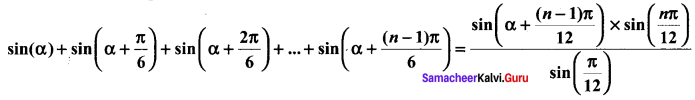Solution: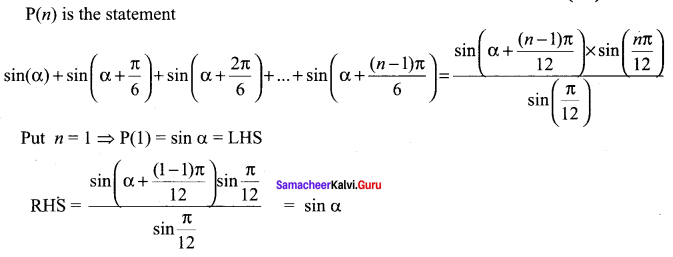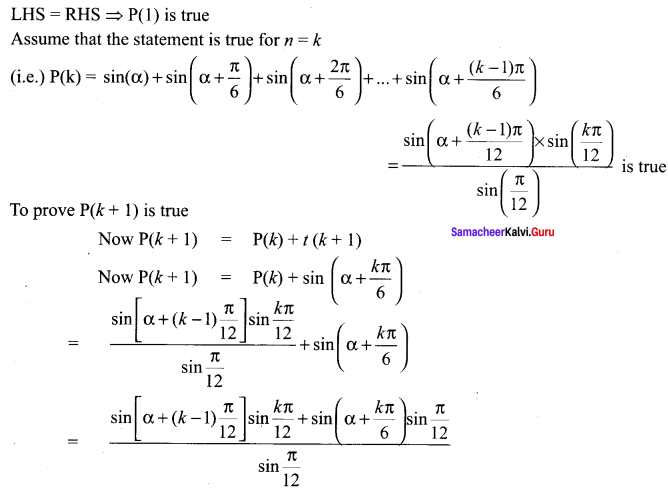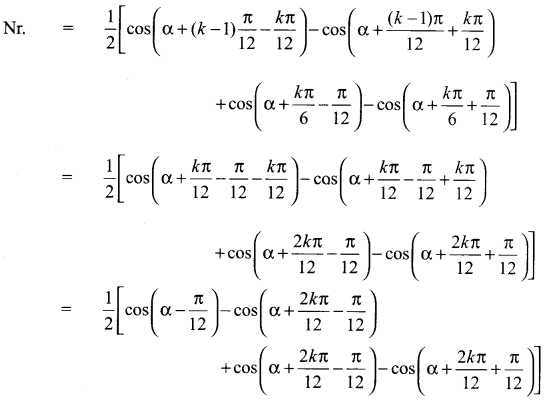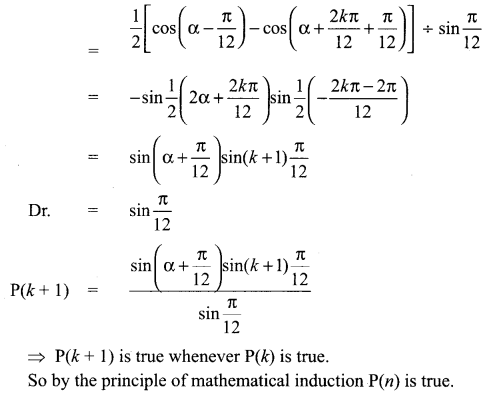### Samacheer Kalvi 11th Maths Solutions Chapter 4 Combinatorics and Mathematical Induction Ex 4.4 Additional Questions

Question 1.
Prove by induction the inequality (1 + x)n ≥ 1 + nx, whenever x is positive and n is a positive integer.
Solution:
P(n) : (1 +x)n ≥ 1 +nx
P(1): (1 + x)1 ≥ 1 + x
⇒ 1 + x ≥ 1 + x, which is true.
Hence, P(1) is true.
Let P(k) be true
(i.e.) (1 + x)k ≥ 1 + kx
We have to prove that P(k + 1) is true.
(i.e.) (1 + x)k + 1 ≥ 1 + (k + 1)x
Now, (1 + x)k + 1 ≥ 1 + kx [∵ p(k) is true]
Multiplying both sides by (1 + x), we get
(1 + x)k(1 + x) ≥ (1 + kx)(1 + x)
⇒ (1 + x)k + 1 ≥ 1 + kx + x + kx2
⇒ (1 + x)k + 1 ≥ 1 + (k + 1)x + kx2 ….. (1)
Now, 1 + (k + 1) x + kx2 ≥ 1 + (k + 1)x …… (2)
[∵ kx2 > 0]
From (1) and (2), we get
(1 + x)k + 1 ≥ 1 + (k + 1)x
∴ P(k + 1) is true if P(k) is true.
Hence, by the principle of mathematical induction, P(n) is true for all values, of n.

Question 2.
32n – 1 is divisible by 8.
Solution:
P(n) = 32n – 1 is divisible by 8
For n = 1, we get
P(1) = 32.1 – 1 = 9 – 1 = 8
P(1) = 8, which is divisible by 8.
Let P(n) be true for n = k
P(k) = 32k – 1 is divisible by 8 ….. (1)
Now, P(k + 1) = 3(2k + 2) – 1 = 32k.32 – 1
= 32(32k – 1) + 8
Now, 32k – 1 is divisible by 9. [Using (1)]
∴ 32 (32k – 1) + 8 is also divisible by 8.
Hence, 32n – 1 is divisible by 8 ∀ n E NQuestion 3.
Prove by the principle of mathematical induction if x and y are any two distinct integers, then xn – yn is divisible by x – y. [OR]
xn – yn is divisible by x – y, where x – y ≠ 0.
Solution:
Let the given statement be P(n).
(i.e.) P(n): xn – yn = M(x – y), x – y ≠ 0

Step I.
When n = 1,
xn – yn = x – y = M(x – y) ….(1)
⇒ P(1) is true.

Step II.
Assume that P(k) is true.
(i.e.) xk – yk = M(x – y), x – y ≠ 0
We shall now show that P(k + 1) is true
Now, xk + 1 – yk + 1 = xk + 1 – xky + xk + 1y – yk + 1
= xk(x – y) + y(xk – yk)
= xk(x – y) + yM(x – y) [Usng ….. (1)]
= (x – y)(xk – yM)
∴ By the principle of mathematical induction, P(n) is true for all n ∈ N

Question 4.
Prove by the principle of mathematical induction that for every natural number n, 32n + 2 – 8n – 9 is divisible by 8.
Solution:
Let P(n): 32n + 2 – 8n – 9 is divisible by 8.
Then, P(1): 32.1 + 2 – 8.1 – 9 is divisible by 8.
(i.e.) 34 – 8 – 9 is divisible by 8 or 81 – 8 – 9 is divisible by 8
(or) 64 is divisible by 8, which is true.
Suppose P(k) is true, then
P(k) : 32k + 2 – 8k – 9 is divisible by 8
(i.e.) 32k + 2 – 8k – 9 = 8m, where m ∈ N (or)
32k + 2 = 8m + 8k + 9
P(k + 1) is the statement given by, …(1)
P(k + 1) : 32(k + 1) + 2 – 8(k + 1) – 9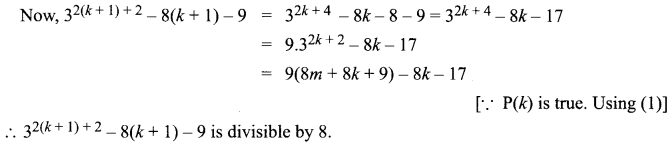∴ P(k + 1) is true
Hence, by the principle of mathematical induction, P(n) is true for all n ∈ N

Question 5.
Use the principle of mathematical induction to prove that for every natural number n.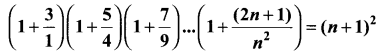Solution:
Let P(n) be the given statement, i.e.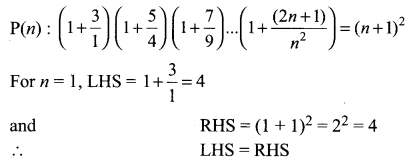⇒ P(1) is true.
We note than P(n) is true for n = 1.
Assume that P(k) is true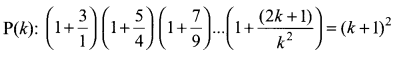Now, we shall prove that P(k + 1) is true whenever P(k) is true. We have,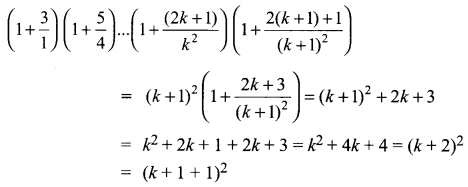∴ P(k + 1) is also true whenever P(k) is true
Hence, by the principle of mathematical induction, P(n) is also true for all n ∈ N.

Question 6.
n3 – n is divisible by 6, for each natural number n ≥ 2.
Solution:
Let P(n) : n3 – n

Step 1 :
P(2): 23 – 2 = 6 which is divisible by 6. So it is true for P(2).

Step 2 :
P(A): k3 – k = 6λ. Let it is be true for k ≥ 2
⇒ k3 = 6λ + k …(i)

Step 3 :
P(k + 1) = (k + 1)3 – (k + 1)
= k3 + 1 + 3k2 + 3k – k – 1 = k3 – k + 3(k2 + k)
= k3 – k + 3(k2 + k) = 6λ + k – k + 3(k2 + k)
= 6λ + 3(k2 + k) [from (i)]
We know that 3(k2 + k) is divisible by 6 for every value of k ∈ N.
Hence P(k + 1) is true whenever P(k) is true.Question 7.
For any natural number n, 7n – 2n is divisible by 5.
Solution:
Let P(n) : 7n – 2n

Step 1 :
P(1) : 71 – 21 = 5λ which is divisible by 5. So it is true for P(1).

Step 2 :
P(k): 7k – 2k = 5λ. Let it be true for P(k)

Step 3 :
P(k + 1) = 7k + 1 – 2k + 1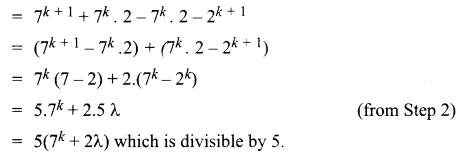So, it is true for P(k + 1)
Hence, P(k + 1) is true whenever P(k) is true.

Question 8.
n2 < 2n, for all natural numbers n ≥ 5.
Solution:
Let P(n) : n2 < 2n for all natural numbers, n ≥ 5

Step 1 :
P(5) : 15 < 25 ⇒ 1 < 32 which is true for P(5)

Step 2 :
P(k): k2 < 2k. Let it be true for k ∈ N

Step 3 :
P(k + 1): (k + 1)2 < 2k + 1
From Step 2, we get k2 < 2k
⇒ k2 < 2k + 1 < 2k + 2k + 1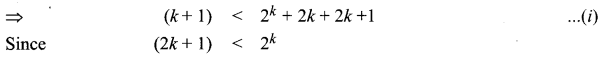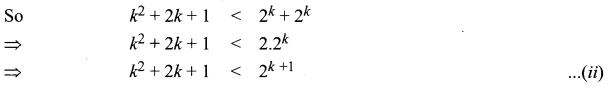From eqn. (i) and (ii), we get (k + 1)2 < 2k + 1
Hence, P(k + 1) is true whenever P(k) is true for k ∈ N, n ≥ 5.

Question 9.
In 2n < (n + 2)! for all natural number n.
Solution:
Let P(n) : 2n < (n + 2)! for all k ∈ N.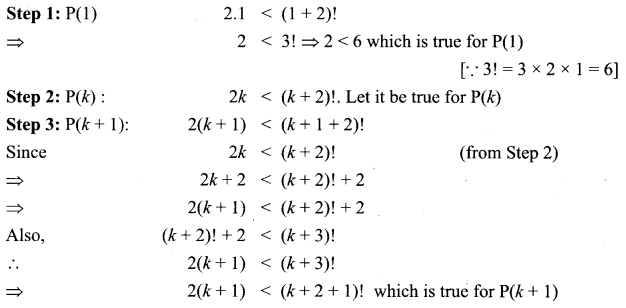Hence, P(k + 1) is true whenever P(k) is true.

Question 10.
1 + 5 + 9 + … + (4n – 3) = n(2n – 1), ∀ n ∈ N.
Solution:
Let P(n) : 1 + 5 + 9 + … + (4n – 3) = n(2n – 1), ∀ n ∈ N

Step 1:
P(1) : 1 = 1(2.1 – 1) = 1 which is true for P(1)

Step 2:
P(k) : 1 + 5 + 9 + … + (4k – 3) = k(2k – 1). Let it be true.

Step 3:
P(k + 1) : 1 + 5 + 9 + … + (4k – 3) = k(4k + 1)
= k(2k – 1) + (4k + 1) = 2k2 – k + 4k + 1
= 2k2 + 3k + 1 = 2k2 + 2k + k + 1
= 2k(k + 1) + 1 (k + 1) = (2k + 1)(k + 1)
= (k+ 1) (2k + 2 – 1) = (k + 1) [2(k + 1) – 1]
Which is true for P(k + 1).
Hence, P(k + 1) is true whenever P(k) is true.